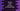# How to iterate and print an array in reverse order in Python## How to print an array in reverse order in Python:

In this post, we will learn how to print an array in reverse order in Python. For example, if the array is [1, 2, 3, 4, 5], it will print it in reverse order i.e. from the last element to the first element:

``5 4 3 2 1``

We will use a loop to do that.

### Algorithm:

The values are stored in sequential order in an array and we can access these elements or values by using the index. The index starts from 0 and ends at length of the array - 1.

i.e. the index of the first element is 0, index of the second element is 1… etc.

To print the elements of the array in reverse, we need to use a loop that will iterate from length - 1 to 0. We can use a for loop or a while loop to write this.

## Method 1: By using a while loop:

Let’s try this with a while loop:

``````given_arr = [1, 2, 3, 4, 5]

i = len(given_arr) - 1

while(i >= 0):
print(given_arr[i])
i = i - 1``````

In this program,

• given_arr is the given array.
• i is the index of the last element of the array. It is equal to length of the array - 1.
• The while loop keeps running while the value of i is equal to or greater than 0.
• Inside the loop, we are printing the element for index i and decrementing the value of i by 1.

If you run this program, it will print the below output:

``````5
4
3
2
1``````

## Method 2: By using a for loop:

We need to use the range function to iterate over the array using a for loop. The range() function returns a sequence of numbers starts from a given number to another given number with a given step.

It is defined as like below:

``range(start, stop, step)``

Where,

• start is the start point of the sequence. By default it is 0 and this is an optional value.
• stop is the stop point of the sequence. It is not included in the sequence. It is not optional.
• step is the step value or difference between each number in the sequence. It is optional and by default it’s value is 1.

If we want to iterate an array in reverse order, we need to create a sequence for the for loop using range with:

• start as length of the array - 1
• stop as -1, it will create the sequence up to 0.
• step as -1 because we are decrementing the values.

Below is the complete program:

``````given_arr = [1, 2, 3, 4, 5]

for i in range(len(given_arr) - 1, -1, -1):
print(given_arr[i])
i = i - 1``````

It will print:

``````5
4
3
2
1``````

Here, the range method returns a sequence from len(given_arr) - 1 to 0 by decrementing 1 for each value.